# McGraw Hill Math Grade 4 Chapter 2 Lesson 5 Answer Key Subtracting Whole Numbers

Practice the questions of McGraw Hill Math Grade 4 Answer Key PDF Chapter 2 Lesson 5 Subtracting Whole Numbers to secure good marks & knowledge in the exams.

## McGraw-Hill Math Grade 4 Answer Key Chapter 2 Lesson 5 Subtracting Whole Numbers

Subtract

Question 1.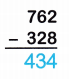Explanation:
Difference between 762 and 328:
762 – 328 = 434.

Question 2.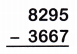Explanation:
Difference between 8,295 and 3,667:
8,295 – 3,667 = 4,628.

Question 3.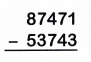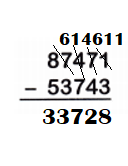Explanation:
Difference between 87,471  and 53,743:
87,471 – 53,743 = 33,728.

Question 4.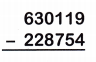Explanation:
Difference between 630,119 and 228,754:
630,119 – 228,754 = 401,365.

Question 5.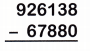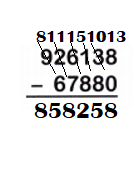Explanation:
Difference between 926,138 and 67,880:
926,138 – 67,880 = 858,258.

Question 6.Explanation:
Difference between 725,914 and 699,311:
725,914 – 699,311 = 26,603.

Question 7.
238,960 – 729 = ___________
Difference between 238,960 – 729 we get 238,231.

Explanation:
238,960 – 729 = 238,231.

Question 8.
46,008 — 19,725 = _____
Difference between 46,008 and 19,725 we get 26,283.

Explanation:
46,008 – 19,725 = 26,283.

Question 9.
52,937 — 8,658 = ___________
Difference between 52,937 and 8,658 we get 44,279.

Explanation:
52,937 – 8,658 = 44,279.

Question 10.
783,209 — 97,120 = ____
Difference between 783,209 and 97,120 we get 686,089.

Explanation:
783,209 – 97,120 = 686,089.

Question 11.
Look at problem 6. Why was the 0 in the hundred thousands place not written ¡n the answer?